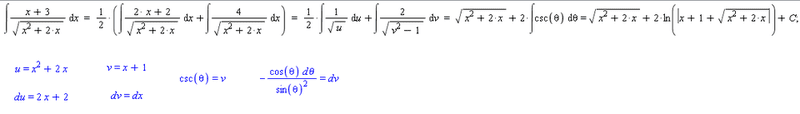# Integral containing something over the root of x²-a²

## Homework Statement

Evaluate the next integrals, expressing it previously in the forms that contains $$\sqrt[ ]{a^2+u^2}$$, $$\sqrt[ ]{a^2-u^2}$$ and $$\sqrt[ ]{u^2-a^2}$$, and then solve it using the integral table;

The exercise which I couldn't solve:

$$\displaystyle\int_{}^{}\displaystyle\frac{x+3}{\sqrt[ ]{x^2+2x}}dx$$

## The Attempt at a Solution

I started by completing the square:

$$x^2+2x=(x+1)^2-1$$

Then

$$u^2=(x+1)^2$$
$$u=(x+1)\Rightarrow{x+3=u+2}$$
$$du=dx$$

$$\displaystyle\int_{}^{}\displaystyle\frac{x+3}{\sqrt[ ]{x^2+2x}}dx=\displaystyle\int_{}^{}\displaystyle\frac{u+2}{\sqrt[ ]{u^2-1}}du$$

From here I've tried to solve it by parts
$$t=u+2$$
$$dt=du$$

$$dv=\displaystyle\frac{1}{\sqrt[ ]{u^2-1}}du$$
$$v=\ln|u+\sqrt[ ]{u^2-1}|$$

$$\displaystyle\int_{}^{}\displaystyle\frac{u+2}{\sqrt[ ]{u^2-1}}du=(u+2)\ln|u+\sqrt[ ]{u^2-1}|-\displaystyle\int_{}^{}\ln|u+\sqrt[ ]{u^2-1}|du$$

If I try again by parts with $$-\displaystyle\int_{}^{}\ln|u+\sqrt[ ]{u^2-1}|du$$ it gets more complicated.

So, what do you say?

Bye there.

## Answers and Replies

Dick
Science Advisor
Homework Helper
You can do integral u/sqrt(u^2-1) with a simple substitution. So split (u+2)/sqrt(u^2-1) up into two integrals.

hunt_mat
Homework Helper
The integral
$$\int\frac{u+2}{\sqrt{u^{2}-1}}du$$
Can be computed by the substitution
$$u=\cosh v$$
Everything should come out in the wash then

Thank you both.

This is what I wasn't seeing:
You can do integral u/sqrt(u^2-1) with a simple substitution. So split (u+2)/sqrt(u^2-1) up into two integrals.

Bye there!

I've arrived to this solution:

$$\displaystyle\int_{}^{}\displaystyle\frac{x+3}{\sqrt[ ]{x^2+2x}}dx=\sqrt[ ]{(x+1)^2-1}+2\ln|x+1+\sqrt[ ]{(x+1)^2-1}|+C$$

Dick
Science Advisor
Homework Helper
I've arrived to this solution:

$$\displaystyle\int_{}^{}\displaystyle\frac{x+3}{\sqrt[ ]{x^2+2x}}dx=\sqrt[ ]{(x+1)^2-1}+2\ln|x+1+\sqrt[ ]{(x+1)^2-1}|+C$$

Seems ok to me. I would write x^2+2*x instead of (x+1)^2-1. But that's up to you.

Thanks Dick. BTW nice nickname :D

yup, got the same answer !P.S: anyone knows a program that writes LaTeX and has an intuitive interface? :)

yup, got the same answer !P.S: anyone knows a program that writes LaTeX and has an intuitive interface? :)

Try this site, I use it frequently :)

http://www.codecogs.com/latex/eqneditor.php